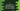# Use pointer to find the largest of three numbers in C# Introduction :

Pointer variables are used to store the address of a variable in C. We can also access these values stored in the address. In this tutorial, we will learn how to find the largest among three numbers using a pointer. This is a beginner level tutorial and you will learn how to read values and store their address in a pointer, how to access values using a pointer variable and how to use if-else loops in C. The program will ask the user to enter three values one by one. It will save the address of three variables in three pointer variables and read the user input values to these variables. Finally, it will find out the largest number using one if-else and print it out to the user.

## C program :

Below is the C program :

``````#include <stdio.h>

int main()
{
//1
int firstNo;
int secondNo;
int thirdNo;
int largestNo;

//2
int *firstPointer = &firstNo;
int *secondPointer = &secondNo;
int *thirdPointer = &thirdNo;

//3
printf("Enter the first number : ");
scanf("%d", firstPointer);

printf("Enter the second number : ");
scanf("%d", secondPointer);

printf("Enter the third number : ");
scanf("%d", thirdPointer);

//4
if (*firstPointer > *secondPointer)
{
//5
largestNo = *firstPointer > *thirdPointer ? *firstPointer : *thirdPointer;
}
else
{
//6
largestNo = *secondPointer > *thirdPointer ? *secondPointer : *thirdPointer;
}

//7
printf("The largest number is : %d\n", largestNo);
}
``````

### Explanation :

The commented numbers in the above program denote the step numbers below :

1. Create three integer variables to store the user input values. Create one integer variable largestNo to hold the largest of the three numbers.

2. Create three integer pointer variables. Assign the address of the variables to these pointer variables. These pointer variables are holding the address of the three integer variables.

3. Ask the user to enter the first, second and third numbers. Store these numbers using the pointer variables. Actually, it is storing these values in the variables firstNosecondNo and thirdNo. The program is using the pointer variables to store them.

4. Use one if-else block to find out the largest number. The if block is checking if the first number is greater than the second number or not.

5. If the first number is greater than the second number, find if the third number is greater than the first number or not. Update the largestNo variable accordingly.

6. If the first number is less than the second number, update the largest number variable similarly.

7. Finally, print the largest number variable i.e. largestNo.

### Sample output :

``````Enter the first number : 1
Enter the second number : 3
Enter the third number : 2
The largest number is : 3

Enter the first number : 3
Enter the second number : 2
Enter the third number : 1
The largest number is : 3

Enter the first number : 19
Enter the second number : 12
Enter the third number : 11
The largest number is : 19
``````

### Conclusion :

In this tutorial, we have learned how to find the largest of three numbers using pointers. Pointers are useful in many cases. Normally, for simple use cases like this, you can use variables instead of pointers. Try to go through the example and drop one comment below if you have any queries.